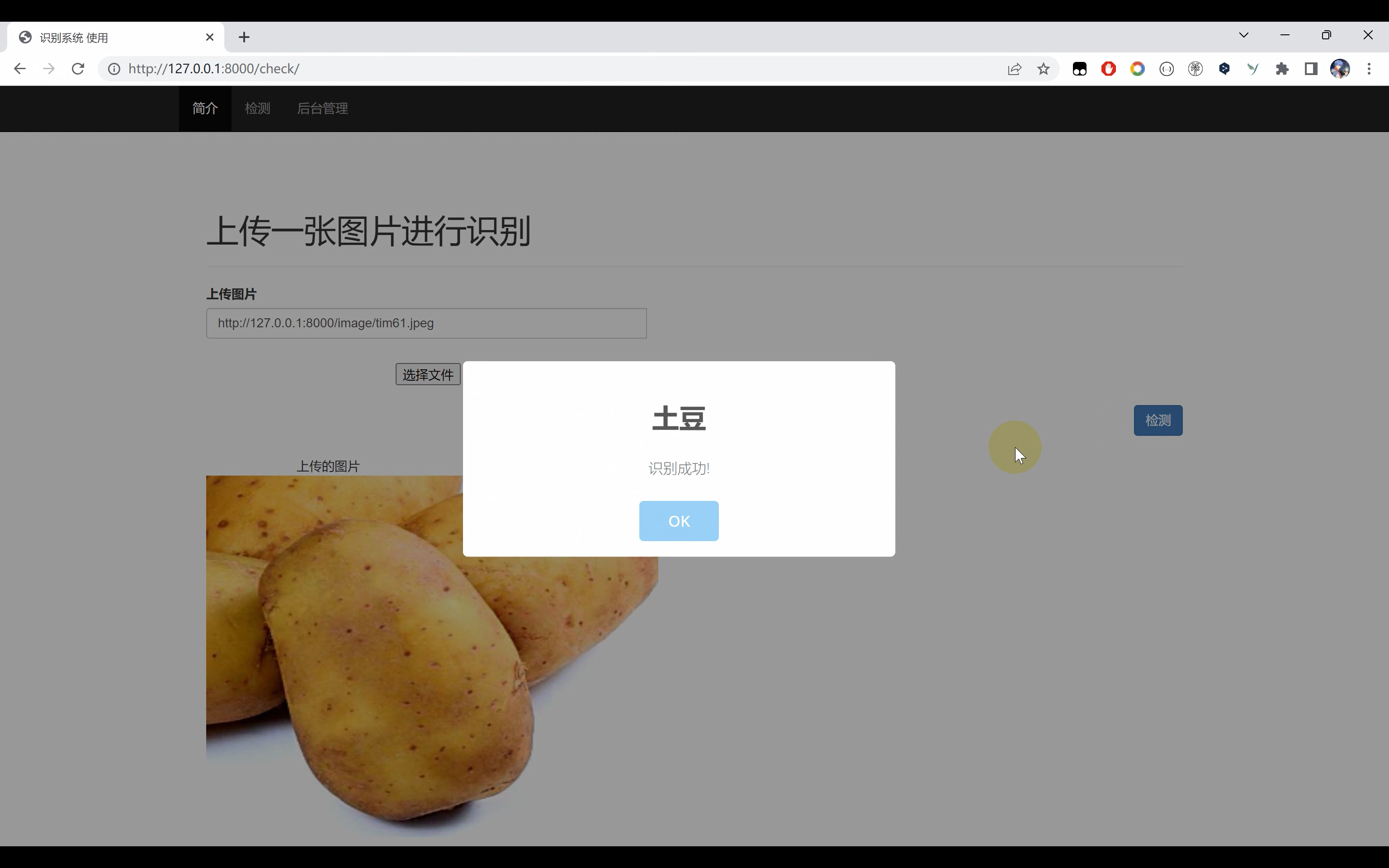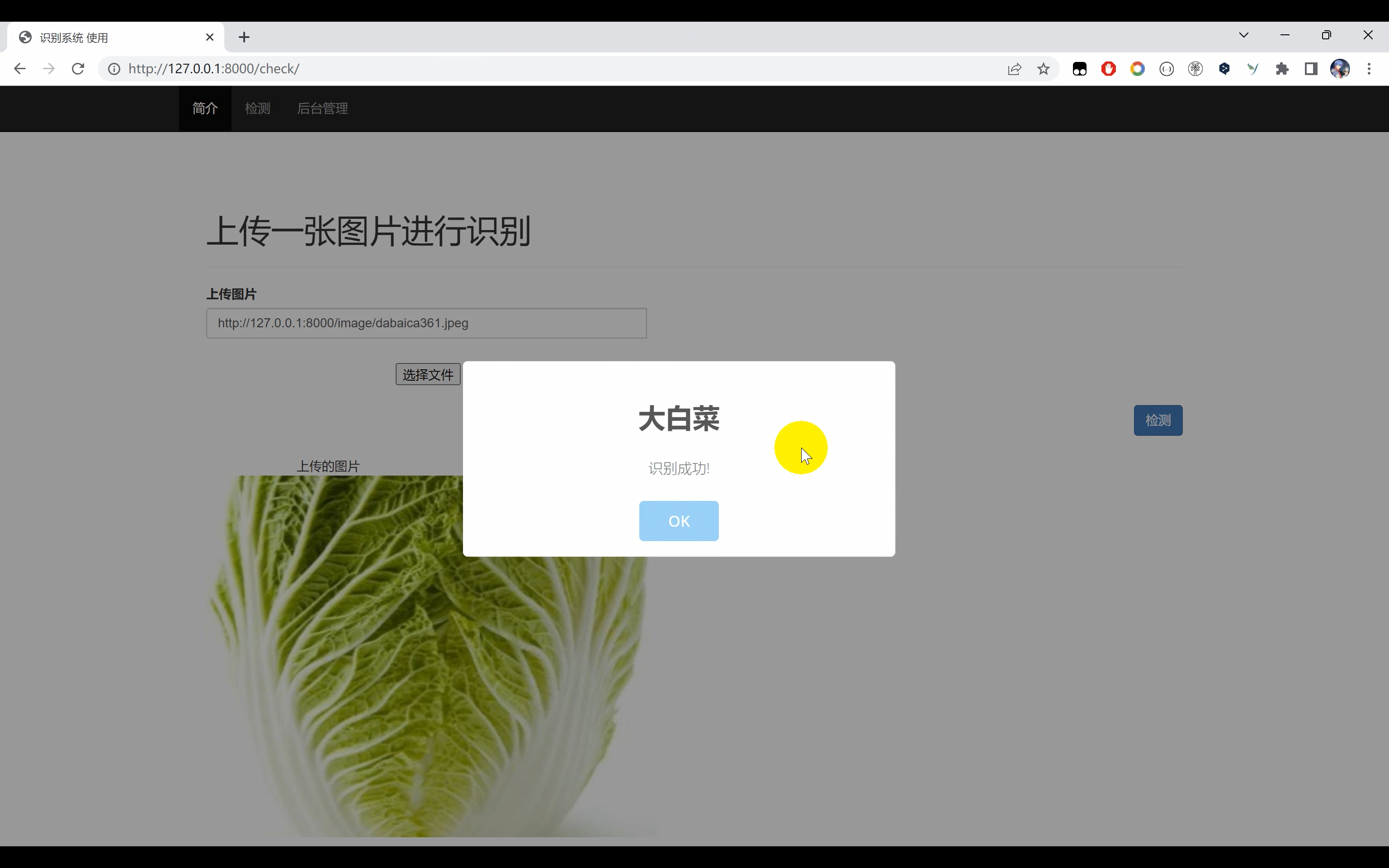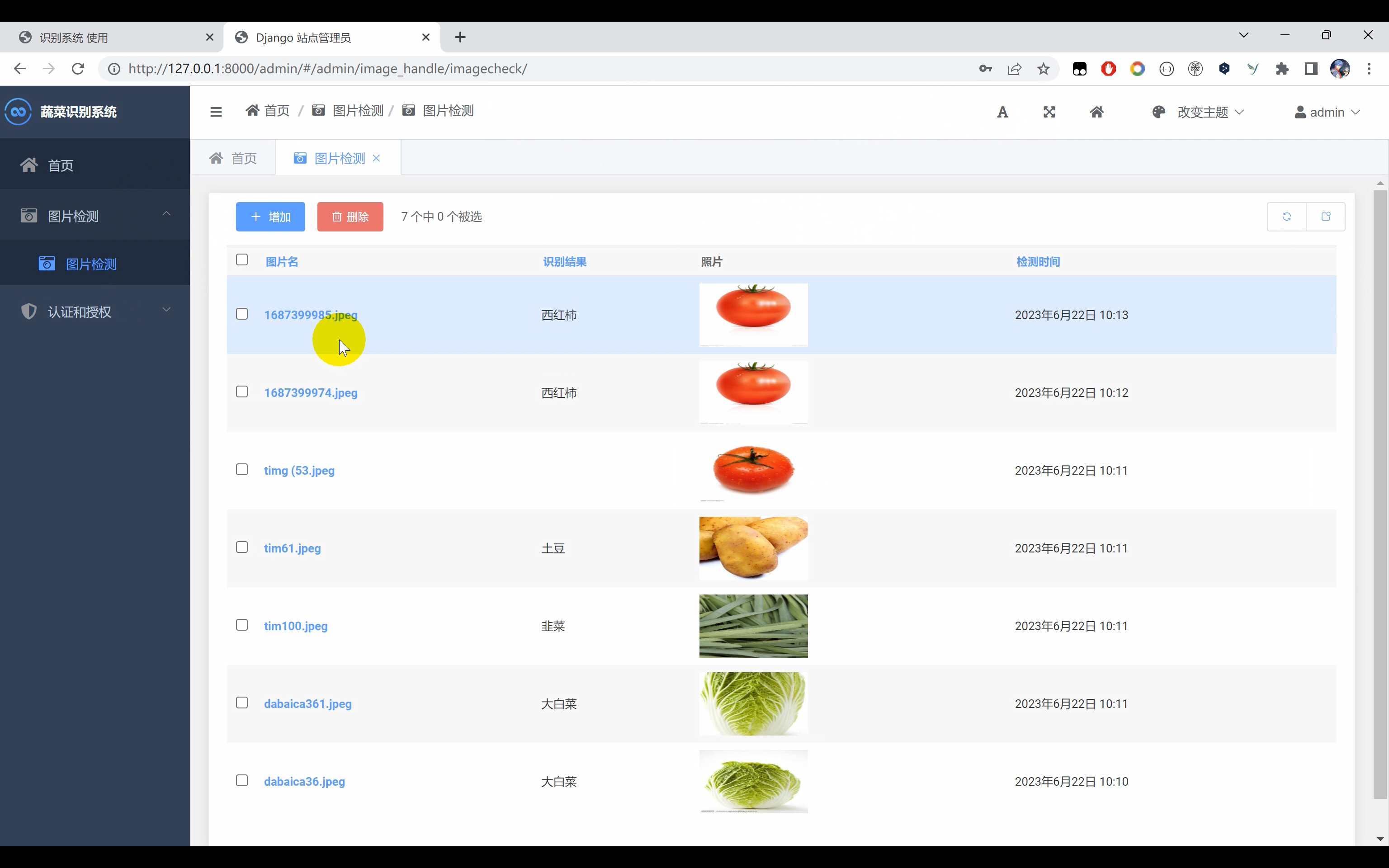# 蔬菜识别系统python+TensorFlow+Django网页界面+卷积网络算法+深度学习模型

+关注继续查看

## 二、效果图片## 四、TensorFlow图像分类示例

import tensorflow as tf
from tensorflow.keras.preprocessing.image import ImageDataGenerator

# 定义数据集路径
train_dir = 'train/'
val_dir = 'validation/'

# 设置图像预处理参数
train_datagen = ImageDataGenerator(rescale=1./255,
rotation_range=20,
width_shift_range=0.2,
height_shift_range=0.2,
shear_range=0.2,
zoom_range=0.2,
horizontal_flip=True)

val_datagen = ImageDataGenerator(rescale=1./255)

# 加载训练数据集
train_generator = train_datagen.flow_from_directory(train_dir,
target_size=(224, 224),
batch_size=32,
class_mode='categorical')

# 加载验证数据集
val_generator = val_datagen.flow_from_directory(val_dir,
target_size=(224, 224),
batch_size=32,
class_mode='categorical')


from tensorflow.keras.applications import VGG16
from tensorflow.keras.models import Sequential
from tensorflow.keras.layers import Dense, Flatten

# 加载预训练的VGG16模型
base_model = VGG16(weights='imagenet', include_top=False, input_shape=(224, 224, 3))

# 冻结预训练模型的权重
for layer in base_model.layers:
layer.trainable = False

# 构建分类模型
model = Sequential()

# 编译模型
loss='categorical_crossentropy',
metrics=['accuracy'])|
18天前
|

24 0
|
27天前
|

14 0
|
2月前
|

29 0
|
2月前
|

57 0
|
2月前
|

OpenCV-图像着色（采用DNN模块导入深度学习模型）
OpenCV-图像着色（采用DNN模块导入深度学习模型）
30 0
|
2月前
|

【深度学习】实验12 使用PyTorch训练模型
【深度学习】实验12 使用PyTorch训练模型
31 0
|
2月前
|

|
3月前
|

50 0
|
3月前
|

24 0
|
4月前
|

40 0